2016-12-07

Mixture model estimation as hypothesis testing

Although I am on the more progressive side of the Statistic philosophy spectrum (avoid NHST and p-value at all cost), sometimes hypothesis testing and its related concepts are still useful. Recently I came across Christian Robert's Blog post and his response to a X validated question, which introduces me to a novel methods of Bayesian hypothesis testing by estimating a mixture model.

The following code is based on the BEST example of PyMC3.

First, generate some normally distributed random data.

# drug = (101,100,102,104,102,97,105,105,98,101,100,123,105,103,100,95,102,106,
#         109,102,82,102,100,102,102,101,102,102,103,103,97,97,103,101,97,104,
#         96,103,124,101,101,100,101,101,104,100,101)
# placebo = (99,101,100,101,102,100,97,101,104,101,102,102,100,105,88,101,100,
#            104,100,100,100,101,102,103,97,101,101,100,101,99,101,100,100,
#            101,100,99,101,100,102,99,100,99)

Nsbj = 42
drug    = np.random.normal(loc=101.5,scale=1,size=Nsbj)
placebo = np.random.normal(loc=100.5,scale=1,size=Nsbj)

y1 = np.array(drug)
y2 = np.array(placebo)
y = pd.DataFrame(dict(value=np.r_[y1, y2],
group=np.r_[['drug']*len(drug),
['placebo']*len(placebo)]))

y.hist('value', by='group');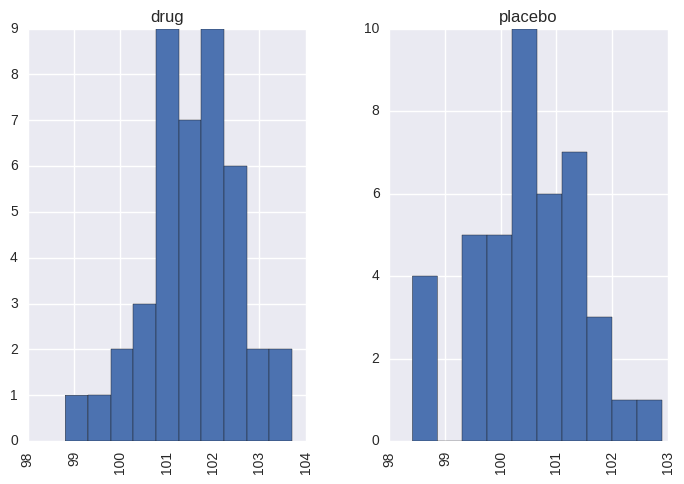The "Bayesian estimation supersedes the t-test" model in PyMC3

# Bayesian Estimation Supersedes the T-Test (BEST) model
μ_m = y.value.mean()
μ_s = y.value.std() * 2
σ_low  = 0
σ_high = 10

with pm.Model() as model:
group1_mean = pm.Normal('group1_mean', μ_m, sd=μ_s)
group2_mean = pm.Normal('group2_mean', μ_m, sd=μ_s)

group1_std = pm.Uniform('group1_std', lower=σ_low, upper=σ_high)
group2_std = pm.Uniform('group2_std', lower=σ_low, upper=σ_high)

group1 = pm.Normal('drug', mu=group1_mean, sd=group1_std, observed=y1)
group2 = pm.Normal('placebo', mu=group2_mean, sd=group2_std, observed=y2)

diff_of_means = pm.Deterministic('difference of means', group1_mean-group2_mean)
diff_of_stds = pm.Deterministic('difference of stds', group1_std-group2_std)
effect_size = pm.Deterministic('effect size',
diff_of_means/np.sqrt((group1_std**2+group2_std**2)/2))

trace = pm.sample(2000, init=None, njobs=2)

pm.plot_posterior(trace[100:],
varnames=['group1_mean', 'group2_mean', 'group1_std', 'group2_std'],
color='#87ceeb');

Assigned NUTS to group1_mean
Assigned NUTS to group2_mean
Assigned NUTS to group1_std_interval_
Assigned NUTS to group2_std_interval_
100%|██████████| 2000/2000 [00:04<00:00, 406.08it/s]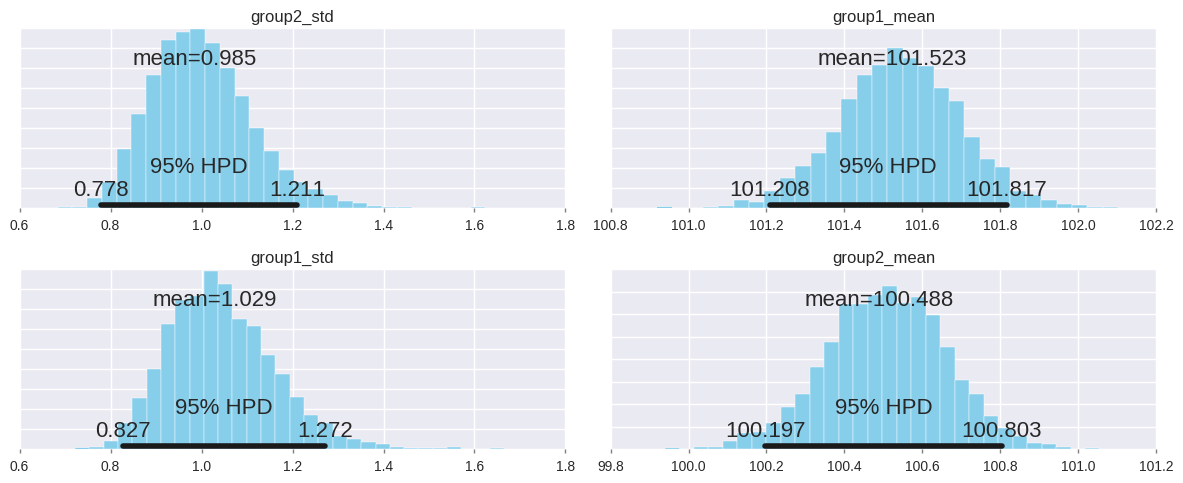As shown above, the model returns pretty accurate paramter estimations using Bayesian Statistics. We can then applied null hypothesis testings with a Bayesian approach using the posterior of the corresponding parameters:

pm.plot_posterior(trace[1000:],
varnames=['difference of means', 'difference of stds', 'effect size'],
ref_val=0,
color='#87ceeb');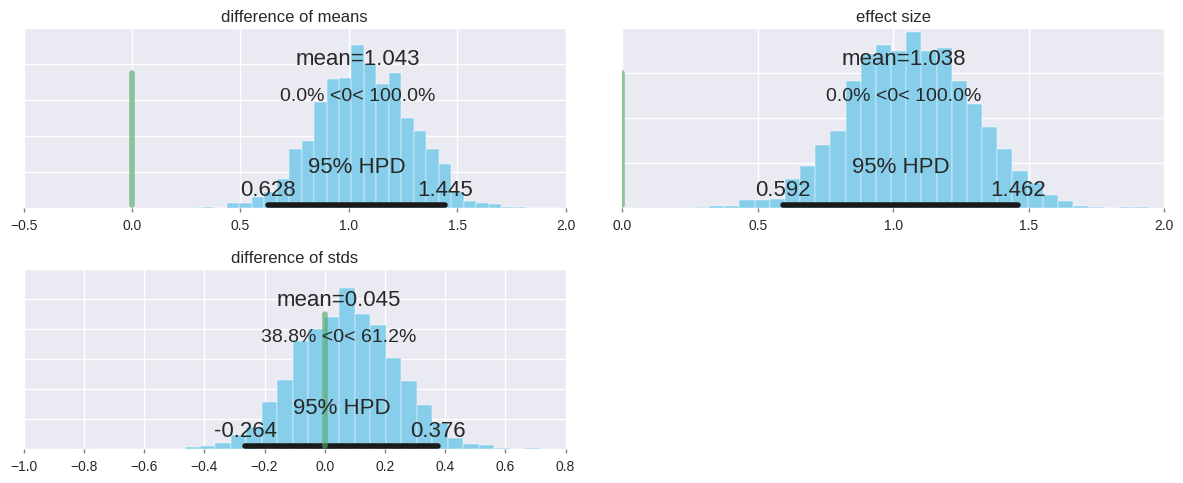This is the approach suggested in Kruschke (2011).

In Kamary et al (2014) they introduced a novel paradigm for Bayesian testing of hypotheses and Bayesian model comparison. The proposed alternative to the traditional construction of Bayes factors or posterior probabilities of a model given the data is to consider the hypotheses or models under comparison as components of a mixture model. Therefore, the original testing problem is replaced with an estimation one that focuses on the probability or weight of a given hypothesis within the mixture model.

A reference $\text{Beta}(a_0,a_0)$ prior on the mixture weights can be used for the common problem of testing two contrasting hypotheses or models. As explained in Kamary et al (2014) "the sensitivity of the posterior estimates of the weights to the choice of $a_0$ vanishes as the sample size increases." Kamary et al advocate a default choice of $a_0 = 0.5$, derived from Rousseau and Mengersen (2011)

# Hypotheses testing via mixture model estimation
import theano.tensor as tt
from pymc3.math import logsumexp
# from pymc3.dist_math import logpow, gammaln

with pm.Model() as model2:
#H0
group0_mean = pm.Normal('group0_mean', μ_m, sd=μ_s)
group0_std = pm.Uniform('group0_std', lower=σ_low, upper=σ_high)

group1_h0 = pm.Normal('drug_h0',    mu=group0_mean, sd=group0_std)
group2_h0 = pm.Normal('placebo_h0', mu=group0_mean, sd=group0_std)

#H1
group1_mean = pm.Normal('group1_mean', μ_m, sd=μ_s)
group2_mean = pm.Normal('group2_mean', μ_m, sd=μ_s)

group1_std = pm.Uniform('group1_std', lower=σ_low, upper=σ_high)
group2_std = pm.Uniform('group2_std', lower=σ_low, upper=σ_high)

group1_h1 = pm.Normal('drug_h1', mu=group1_mean, sd=group1_std)
group2_h1 = pm.Normal('placebo_h1', mu=group2_mean, sd=group2_std)

a = pm.Dirichlet('mixing',a=np.ones((2))*.5)
group1 = pm.Mixture('drug',    w=a,
comp_dists=[group1_h0.distribution,
group1_h1.distribution], observed=y1)
group2 = pm.Mixture('placebo', w=a,
comp_dists=[group2_h0.distribution,
group2_h1.distribution], observed=y2)
#     a = pm.Beta('mixing',alpha=.5,beta=.5)
#     def mixturelogp(w,dist1,dist2):
#         logp1 = dist1.distribution.logp
#         logp2 = dist2.distribution.logp
#         def logp_(value):
#             logps = tt.log(w) + logp1(value) + tt.log(1-w) + logp2(value)
#             return tt.sum(logsumexp(logps))
#         return logp_
#     group1 = pm.DensityDist('drug',    mixturelogp(a,group1_h0,group1_h1),
#                             observed=y1)
#     group2 = pm.DensityDist('placebo', mixturelogp(a,group2_h0,group2_h1),
#                             observed=y2)
start = pm.find_MAP()
trace = pm.sample(2000)

Optimization terminated successfully.
Current function value: 128.025138
Iterations: 23
Function evaluations: 35

Auto-assigning NUTS sampler...
Average ELBO = -133.19: 100%|██████████| 200000/200000 [01:07<00:00, 2945.46it/s]
Finished [100%]: Average ELBO = -133.25
100%|██████████| 2000/2000 [05:34<00:00,  5.97it/s]


Here, I am using the pymc3.Mixture to construct the mixture likelihood function. You could also write your own mixture likelihood function (see the commented part above).

As shown below, the mixture weights heavily favor the alternative hypothesis (mixing_1).

burnin = 200
pm.plot_posterior(trace[burnin:],
varnames=['mixing',
'group0_mean', 'group0_std',
'group1_mean', 'group2_mean', 'group1_std', 'group2_std'],
color='#87ceeb');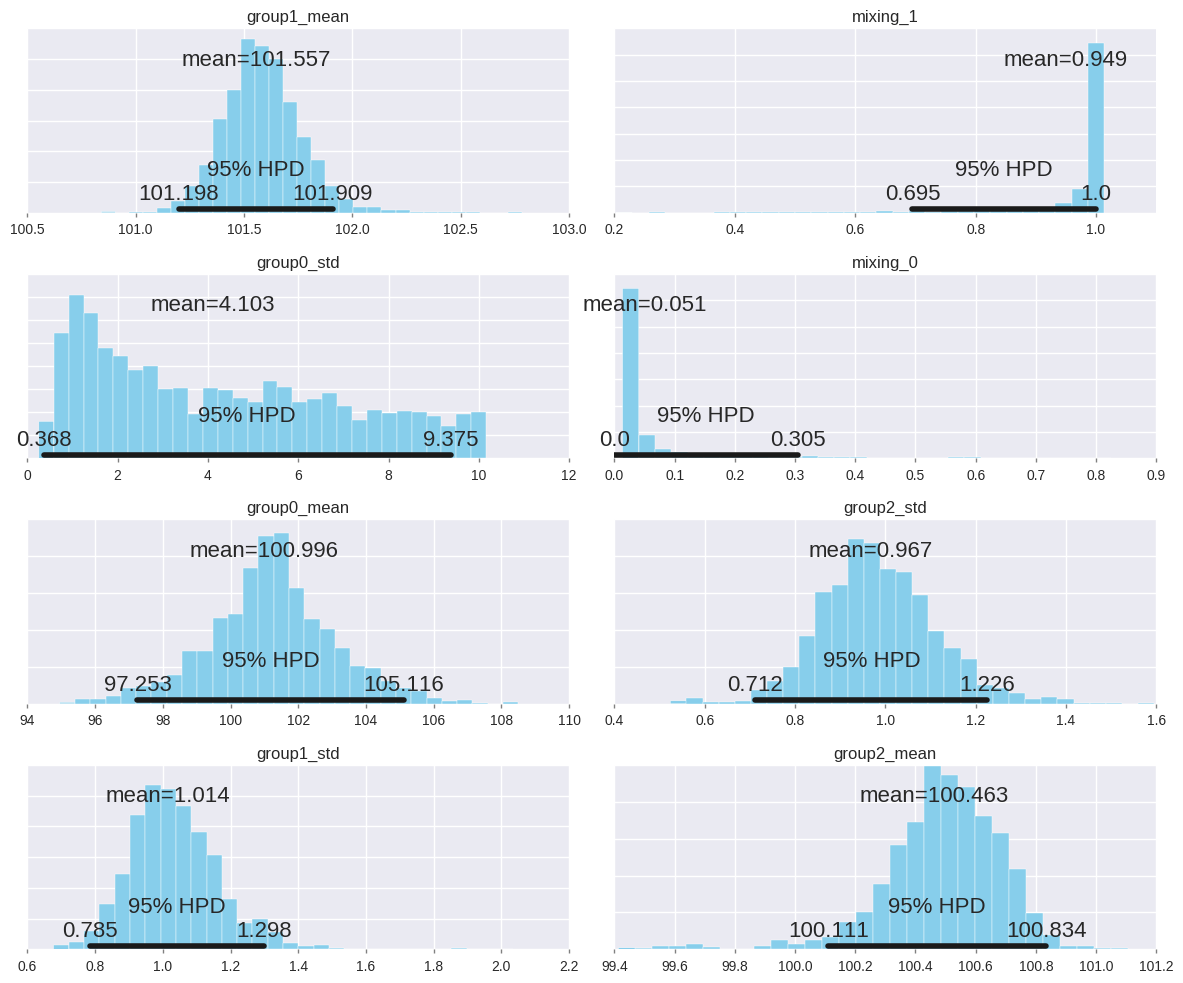import matplotlib.pylab as plt
pm.traceplot(trace[burnin:],
varnames=['mixing',
'group0_mean', 'group0_std',
'group1_mean', 'group2_mean', 'group1_std', 'group2_std'])
plt.show()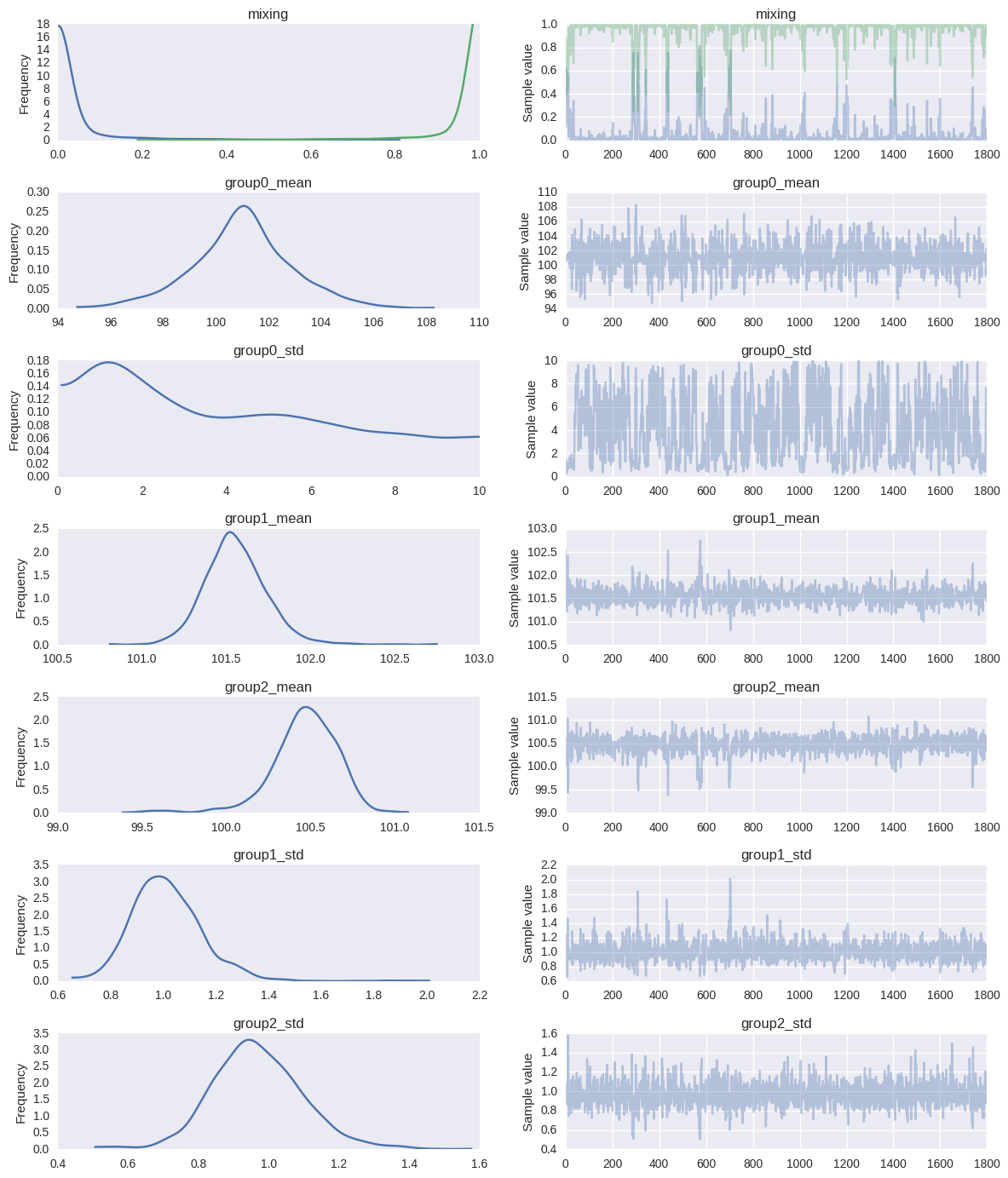The original Jupyter Notebook could be found on Gist.